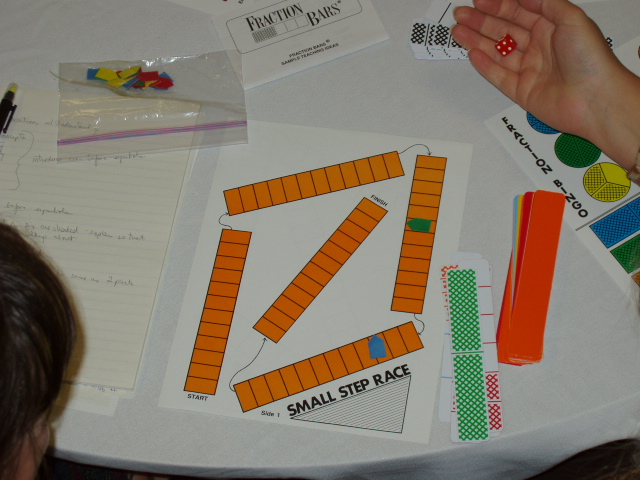Home
 Fractions and Operations The following files contain activities for connecting the fraction concepts introduced at the concrete level in the preceding files to fraction notation and rules for determining equality, inequality, and computing with fractions. Introducing FractionsEquality Inequality Addition and Subtraction Multiplication Divison Roll and Race: Whole number from die times fraction from bar